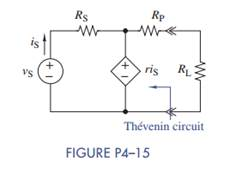Create an Account

Home / Questions / (a) Find the Thévenin equivalent circuit that the load R L sees in Figure 4–15. (b) Then if R P =...

(a) Find the Thévenin equivalent circuit that the load R L sees in Figure 4–15. (b) Then if R P =...

(a) Find the Thévenin equivalent circuit that the load RL sees in Figure 4–15.

(b) Then if RP = r = RL = 10 kΩ, RS = 1kΩ, and vS = 1 V, find the power delivered to the load resistor.Jul 28 2020 View more View LessSubscribe To Get Solution